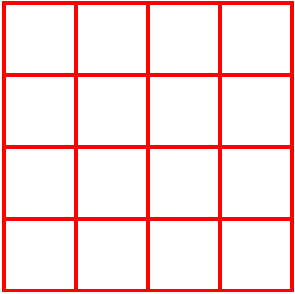13 June 2020

# Really hard puzzle. Can you help me?

I have recently started doing Kenken puzzles (the 6×6 version). Last night, a question came to my mind and ever since I can neither solve the problem nor get it off my mind.

The usual Kenken is a 4×4 grid where you have to fill in every row and every column with the digits 1,2,3 and 4. One rule is that you have to have each one of those digits in every row and every column. (meaning therefore, no repeats in a row or column either – like Sudoku).

There are other rules for each individual Kenken problem. However, assume there is no other rule. You can fill in every row and every column with the digits 1,2,3 and 4 – with the only rule being you have to use each one of them once in every row and every column… how many such combinations are possible?

(I am attaching a picture here of an actual Kenken puzzle – but thatPosted June 13, 2020 by Rajib Roy in category "Puzzles

1.By Harmindar Singh Matharu on

(n-1)! * (n-1)!
where n is the length of a side of the square.
For a 4 by 4 square, it is (4-1)! * (4-1)! = 3! * 3! = 36

2.By Rajib on

Harmindar, I will think about it tomorrow…. too sleepy now….

3.By Rajib on

Harmindar, it does not work for n= 2, right? For a 2×2 square, there two possible orientations.

1.By Harmindar Singh Matharu on

Both of them are same due to Symmetry. Rotate one by 90 degree, you get the other. In my proposed solution I have eliminated duplicates which are achieved by rotations.

4.By Rajib Roy (Post author) on

Harmindar, I did not get the how they are duplicates. In fact, try 3by3. There will be 12 valid answers (including all combinations). While enumerating the 3×3, I realized something – the answer might be n! X (n-1)!

The argument (and I am not totally convinced of it is this)…
The top row can be arranged in n! ways. Each will have some combination that will be a solution. (This point I need to prove)
For any such combination, fix the top row. Think the rest of the rows below as individual entities (each row is an entity). Interchanging each one of those entities (row as a whole) will give independent solutions. That can be done in (n-1)! Ways.

I can argue that any possible combination Of answer will be covered by the above (I think I need a more solid proof for that assertion).

It works for 2 = 2!x1! = 2
It works for 3 = 3!x2! = 6
It should work for 4 = 4!x3! = 144. But I need to enumerate this out.

5.By Matharu on

Take the case of 2 X 2 grid/square:

Start Row exchange
1 2 2 1
2 1 1 2
column exchange
2 1 1 2
1 2 2 1

There are total four combinations having only two unique combinations without rotations. This is given by your formula: n! * (n-1)!

If you rotate the first grid by 90 degrees at a time (total 3 rotations), each of the remaining three combinations can be achieved. In my solution, I considered them to be equivalent and hence the formula (n-1)! * (n-1)! giving (2-1)! * (2-1)! = 1 * 1 = 1

For a 3 X 3 grid, total number of combinations is: 3! * 3! = 36. Number of unique combinations without rotations is: 3! * (3-1)! = 12. Applying equivalence by 90 rotations, number of unique combinations is: (3-1)! * (3-1)! = 4

6.By Matharu on

Better formatting the grids in my reply above.

Start Row exchange
1 2 2 1
2 1 1 2
column exchange
2 1 1 2
1 2 2 1

1.By Harmindar Singh Matharu on

Sorry, formatting gets lost.

1.By Harmindar Singh Matharu on

My formula given above is incorrect.

Correction to my formula: the number of combinations of a grid of size n X n with 90 degree rotations equivalence is given by: n! * (n-1)! / 4
Where 4 is the number of distinct rotations.

This site uses Akismet to reduce spam. Learn how your comment data is processed.